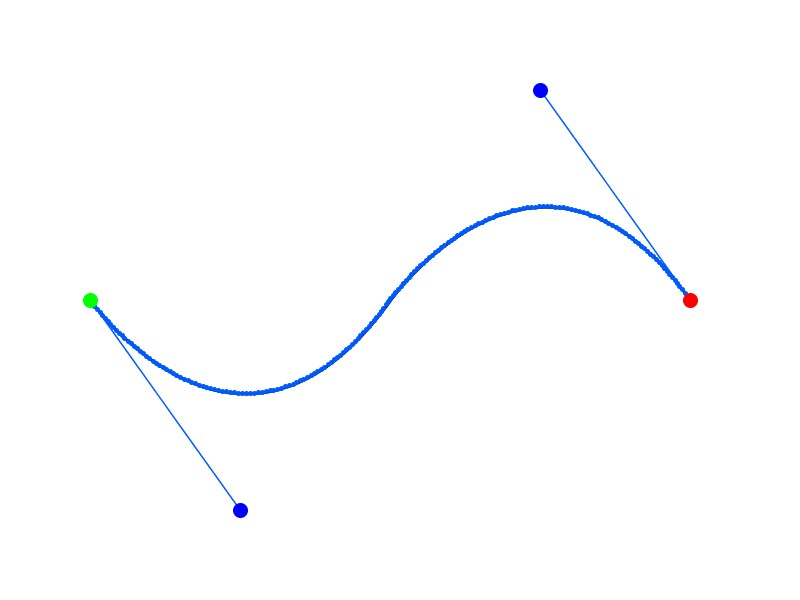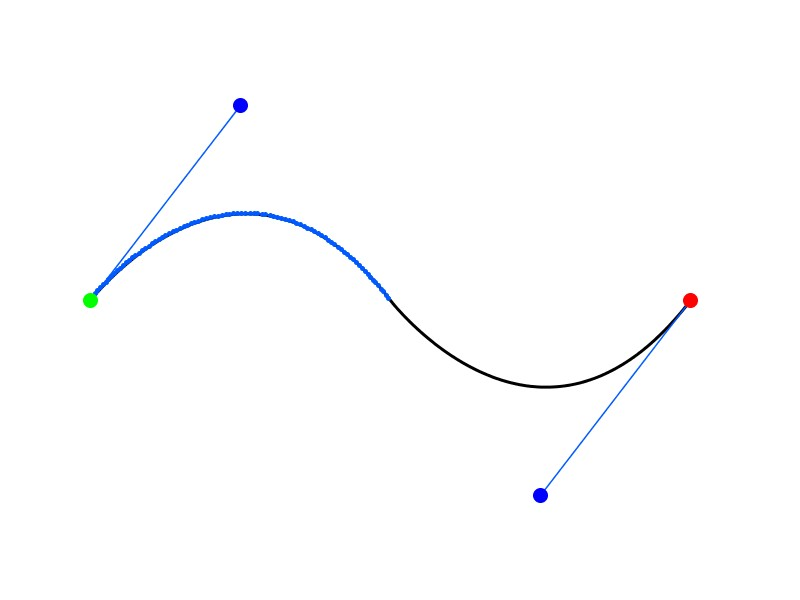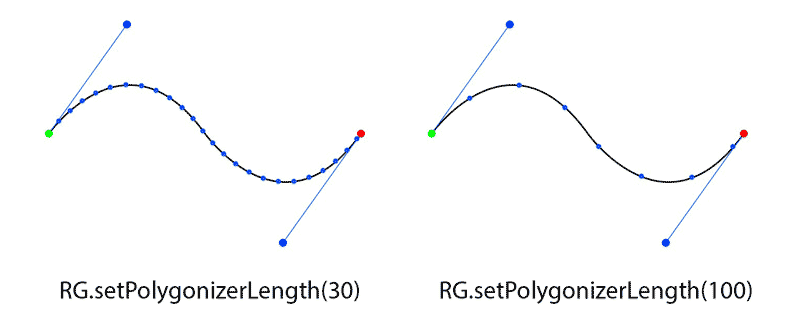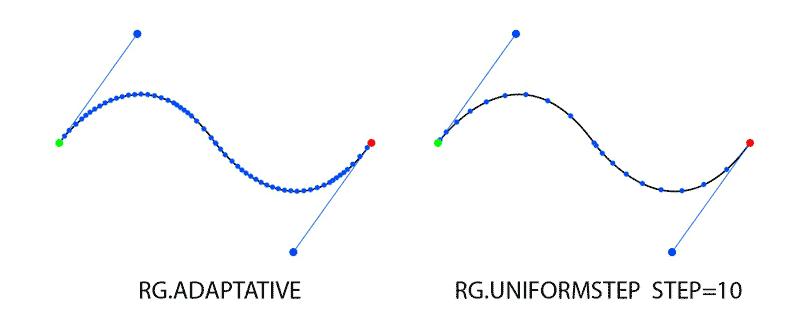# Placing points along an Arc using Geomerative Polygonizer and Processing

/, Programming, Projects, Uncategorized/Placing points along an Arc using Geomerative Polygonizer and Processing

## Placing points along an Arc using Geomerative Polygonizer and Processing

This is a follow on from this post on drawing arcs with Geomerative, a great library for processing (Website) by Ricard Marxer.  I have struggled to find many similar sketches online other than those included in the examples, so i figured I would share my progress. This post whilst keeping a log for my own future use, I hope may serve as a reference for others out there with the same aims as me.

Here we will begin to investigate how we can also place text along these arcs. In order to do so, we will need to establish the coordinates of regularly spaced points along the curve surface. We will also continue on with the same code for familiarity.

First attempt was to figure out exactly what the getPoints()  function within the RShape class retrieves. Once we call this function and place the points into a point array, we can draw each one by simply adding a quick for loop (This method is used in the geomerative tutorial “HelloWorld_GetPoints” with text).Adding blue points at each “point” returned by getPoints() function.

The code to produce this is:

```import geomerative.*;

RShape wave;

float movement=0;
float plusminus = 5.0;
float scale = 3;

//Somwhere to store our curve points
RPoint[] points;

void setup()
{
// From the examples we must always initialise the library using this command
RG.init(this);

//Usual Processing Jazz
size(800 ,600);
background(255);
frameRate(25);
}

void draw(){
//Blank Background each frame
background(255);

//Iterate our movement variable for animation
movement += plusminus;

//Create a new RShape each frame
RShape wave = new RShape();

//At the moment the wave object is empty, so lets add a curve:
wave.addBezierTo(0*scale, 100*scale, 50*scale, (25+movement)*scale, 100*scale, 100*scale);
wave.addBezierTo(100*scale, 100*scale, 150*scale, (175-movement)*scale, 200*scale, 100*scale);

//Lets start to draw away from the edges
translate(30*scale,0*scale);

//Draw our wave
noFill();
stroke(0);
strokeWeight(1*scale);
wave.draw();

//Add some points along the curve
points = wave.getPoints();

stroke(0, 90, 255); //Blue
strokeWeight(5);
for(int i=0; i&amp;amp;lt;points.length; i++){ point(points[i].x, points[i].y); } //Draw our bezier handle lines strokeWeight(0.5*scale); line(0*scale,100*scale,50*scale,(25+movement)*scale); line(150*scale,(175-movement)*scale,200*scale,100*scale); //Finally draw our defined control points strokeWeight(5*scale); stroke(0, 255, 0); //Green point(0*scale,100*scale); stroke(0, 0, 255); //Blue point(50*scale,(25+movement)*scale); point(150*scale,(175-movement)*scale); stroke(255, 0, 0); //Red point(200*scale,100*scale); //If we are at the top of our movement, reverse the directing by //changing the plusminus variable if(movement &amp;amp;gt; 150)
{
plusminus = -5;
}else if( movement &amp;amp;lt;= 0)
{
plusminus = 5;
}

}
```

As the density of points returned by getPoints() is so high, it seems that the whole line is just a badly smoothed blue line. So we can prove to ourselves  this is not the case by quickly plotting half the points:Done by adjusting the bounds of the for loop:

```for(int i=0; i&amp;lt;points.length/2; i++){
point(points[i].x, points[i].y);
}
```

That is far too many points for our requirements, now we could look into just drawing every n-th point but the geomerative example alludes to a better way, manipulating the “Polygonizer”.

## Better understanding the Geomerative “Polygonizer”

Now these values seem to be something we set in the library directly, rather than to the shape objects themselves, implying that this will cause a global change. Lets have a play and see what some of these options do.

First lets take the “UNIFORMLENGTH” setting used in the tutorial, manually defining a length of 30 and 100.

```
RG.setPolygonizer(RG.UNIFORMLENGTH);
RG.setPolygonizerLength(30);

```

The two resultant outputs are:Here is an animation of the UNIFORMLENGTH Polygonizer with different lengths of 30 and 100.

This simple animation works well to show how the UNIFORMLENGTH mode of the polygonizer responds. As the length of the curve increases, further points are added to maintain the specified length between them.

Next a quick look at the alternative modes of:

```RG.setPolygonizer(RG.UNIFORMSTEP);
RG.setPolygonizerStep(10);
```

and

```RG.setPolygonizer(RG.ADAPTATIVE);
```This animation compared the ADAPTIVE and UNIFORMSTEP modes of the polygonizer

The next post will cover using these points to place text along the curve.

By |2016-11-05T11:17:47+00:00June 25th, 2016|Kinetic Storyteller, Programming, Projects, Uncategorized|1 Comment